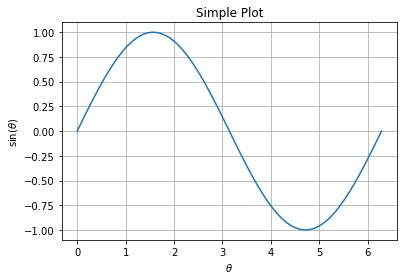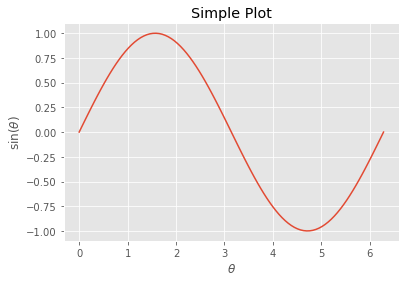--- title: "Plotting with Python" file_title: Plotting ---

This is a MATLAB users introduction to making great plots with Matplotlib in python.

First we need to import the matplotlib.pyplot library, and we can access the functions in that library using the plt namespace, this is a typical style but any prefix works. This allows us to avoid typing matplotlib.pyplt.function() to call functions from that library.

In :
import matplotlib.pyplot as plt


Next, we need the numerical python (numpy) library. This is imported under the namespace np.

In :
import numpy as np


We need some data to plot, so we will use the linspace function of the numpy library to generate a linearly spaced array of values we will use inputs to a function.

In :
theta = np.linspace(0, 2*np.pi, 100)


Now, we need to instatiate figure and axes objects to plot things on to them. Note that there is no need to clear the frame, since a clean slate will always be generated. Also fig and ax are simply a variable choice, I could have just easily said fig1 and ax1, which may happen if we want to make multiple figures.

In :
fig, ax = plt.subplots()
ax.plot(theta, np.sin(theta))
ax.set_title('Simple Plot')
ax.set_xlabel(r'$\theta$')
ax.set_ylabel(r'$\sin(\theta)$')
ax.grid(True)We can use different in-built plot styles to make the style plot looks exactly like we want. The available styles may look different on your system, but you can easily print a list of available plot styles with the following:

In :
for style in plt.style.available:
print(style)

seaborn-ticks
ggplot
dark_background
bmh
seaborn-poster
seaborn-notebook
fast
seaborn
classic
Solarize_Light2
seaborn-dark
seaborn-pastel
seaborn-muted
_classic_test
seaborn-paper
seaborn-colorblind
seaborn-bright
seaborn-talk
seaborn-dark-palette
tableau-colorblind10
seaborn-darkgrid
seaborn-whitegrid
fivethirtyeight
grayscale
seaborn-white
seaborn-deep

In :
plt.style.use('ggplot')
# replot the plot.
fig, ax = plt.subplots()
ax.plot(theta, np.sin(theta))
ax.set_title('Simple Plot')
ax.set_xlabel(r'$\theta$')
ax.set_ylabel(r'$\sin(\theta)$')
ax.grid(True)Now, we can use save the figure as a high quality pdf to include in a LaTeX document, or a png to include in a Word document. The following cell will print out a list of available filetypes.

In :
ext = fig.canvas.get_supported_filetypes().items()
for key, value in ext:
print(f'{key}: {value}')

ps: Postscript
eps: Encapsulated Postscript
pdf: Portable Document Format
pgf: PGF code for LaTeX
png: Portable Network Graphics
raw: Raw RGBA bitmap
rgba: Raw RGBA bitmap
svg: Scalable Vector Graphics
svgz: Scalable Vector Graphics


Now, we can save the figure to the current directory by using the savefig method of the fig object.

In :
fig.savefig('OOP-Plot.pdf',    #name of pdf file
transparent=True,  #make bkgd transparent
bbox_inches='tight'#reduce whitespace
)

In [ ]: